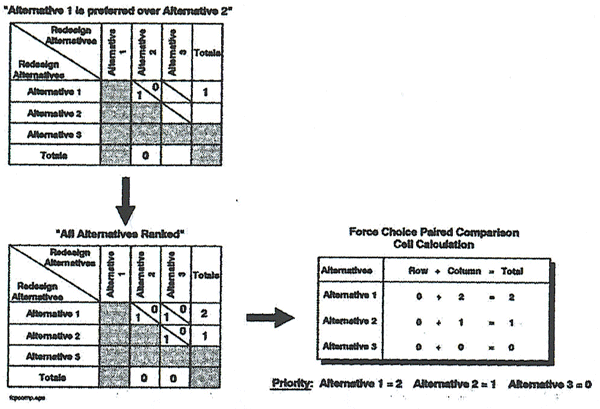Project Management
PMI Sites
Project Management
• Webinars
• Templates
• Community
• Topics
• Knowledge & Tools
• Events
• Other PMI Sites
• PMI Sites

# Force Choice Paired Comparison

 Contents 1 Application 2 Procedures 3 Instructions 4 Example 5 ReferenceA technique to assist in the decision making process. A variation of the decision matrix, choices of recommendations, alternatives, or solutions for reengineering or redesign are arranged as both rows and columns. Participants choose a preference for each possible pair of choices, and the results are tabulated to provide a prioritized result.

This technique is derived from psychological scaling techniques used to determine dominant attributes of behavior and reactions to various stimuli. It has been applied in decision making scenarios over many years.

## Application

• To facilitate consensus-based decision making and problem solving related to the choice of reengineering alternatives.

## Procedures

1. Confirm the issue, goal, or problem being analyzed.
2. List all the choices. Choices can be developed during an activity redesign session or brainstormed while executing this technique.
3. Map the choices on a matrix (see example below).
4. Divide each cell of the matrix in half along the diagonal.
5. Evaluate each choice pair.
6. Add the total choices for each alternative.
7. Review the results and reach consensus.

## Instructions

Confirm the issue, goal, or problem that is being analyzed. Using Brainstorming or another information collection technique, solicit choices from the participants (if alternatives have not yet been determined). It is important to keep the number of choices small when applying this technique because the number of comparisons can grow very large. (For example, if there are 20 choices to be compared, there are 190 possible combinations!) One way to keep the choices down is to simply compare the choices to determine which have similar characteristics. This will help group the choices to enable more effective comparison. During a reengineering project or redesign effort, usually a small number of alternatives need to be evaluated. Force Choice Paired Comparison can be used in conjunction with other forms of analysis such as Cost Benefit Analysis. List the alternative choices (solutions, implementation alternatives, etc.) on the horizontal and vertical axes of the matrix at the top of column and the beginning of each row. This is shown in the example which follows as alternatives 1, 2, and 3. Divide each cell in half along the diagonal. Evaluate, for each pair of choices/alternatives, the group's preference.

It may be helpful to ask the participants: "Which alternative do you prefer, 1 or 2." Place a 1 in the cell half preferred, a 0 in the cell half not chosen. In the first matrix of the example shown below, Alternative 1 is compared to Alternative 2. Alternative 1 is preferred, therefore, a "1" is placed in the bottom half of the cell intersection of Alternatives 1 and 2. Using this methodology, complete comparisons for all choices (Note: No choice is required for an alternative against itself). Add the bottom half of the cells across each row. Add the top half of the cells for each column. In the completed matrix, note that the total for the row Alternative 1 is "2" and the total for the column Alternative 2 is "0". Add the results for each choice (column plus row totals). This is shown in the example for the cell calculation.

In the example which follows, the alternative with the highest ranking priority is Alternative 1 with a total score of 2. Alternative 3 has the lowest total, 0, and is the least preferred. Review the outcome and have the participants reach a consensus and document the results.

## Example## Reference

1. David Meister. Behavioral Analysis and Measurement Methods. John Wiley & Sons, 1985.